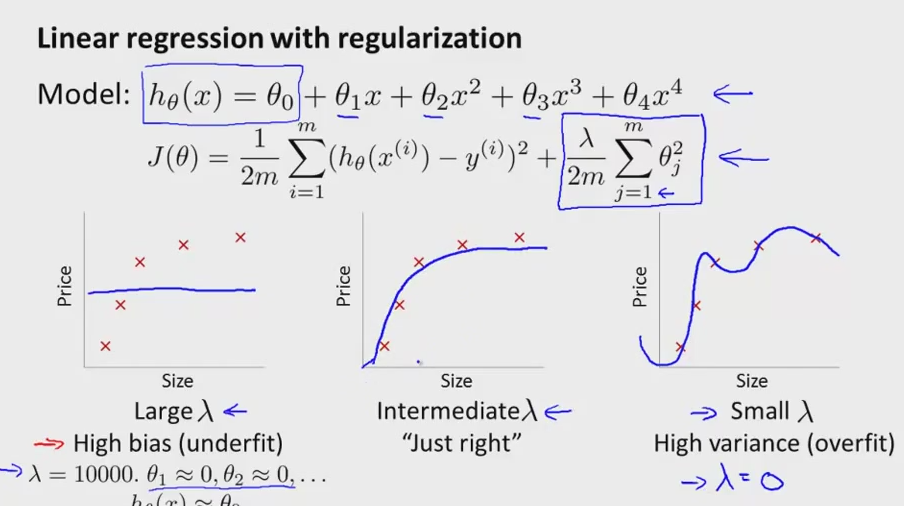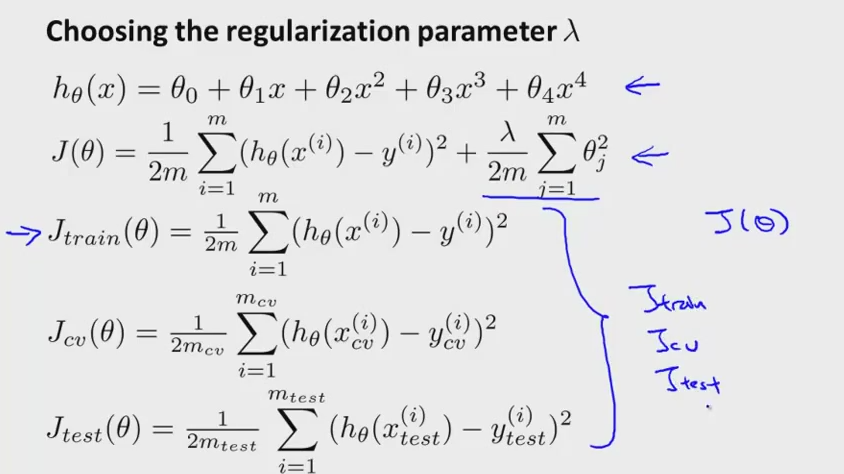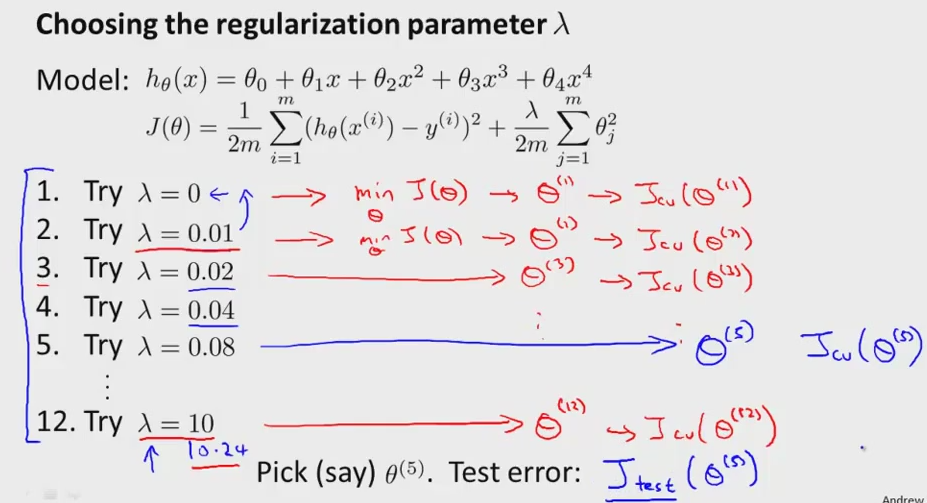• Regularization can avoid underfitting/overfitting. But how it does acttually affect the learning algorithms• Remember the regularization indexes from 1
• Set lambda  = 1000, and each parameters will be highly penalized and will be tend to flat graph, resulting to underfitting
• In contrast, set lambda to 0, the parameters will not be penalized and resulting in overfitting problems
• So how we choose the correct value of regularization (lambda)?• Using extra lambda, just using average of the training set
• Jtrain, Jcv,Jtest in earlier without the regularization• Try variant range of lambda by multiple sets of two
• Iterate each model of theta use it to cost function
• Use the theta into cross validation set
• Pick whichever model that has the lowest value in cross validation error
• And compare it to Jtest error
• Concretely, use the selection model of thetas with selection of lambda,(model 5 with lambda no.5), and pick whichever has the lowest error of Jcv
• That's the summary of model selection for regularization